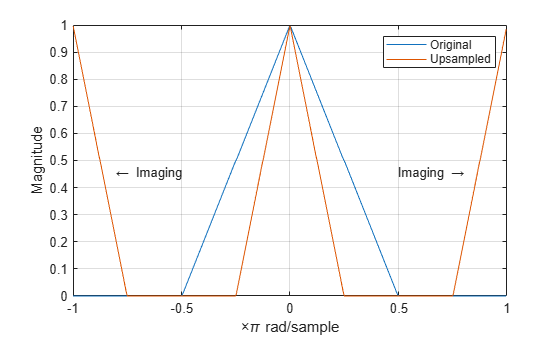# Upsampling — Imaging Artifacts

This example shows how to upsample a signal and how upsampling can result in images. Upsampling a signal contracts the spectrum. For example, upsampling a signal by 2 results in a contraction of the spectrum by a factor of 2. Because the spectrum of a discrete-time signal is $2\pi$-periodic, contraction can cause replicas of the spectrum normally outside of the baseband to appear inside the interval $\left[-\pi ,\pi \right]$.

Create a discrete-time signal whose baseband spectral support is $\left[-\pi ,\pi \right]$. Plot the magnitude spectrum.

f = [0 0.250 0.500 0.7500 1]; a = [1.0000 0.5000 0 0 0]; nf = 512; b = fir2(nf-1,f,a); Hx = fftshift(freqz(b,1,nf,'whole')); omega = -pi:2*pi/nf:pi-2*pi/nf; plot(omega/pi,abs(Hx)) grid xlabel('\times\pi rad/sample') ylabel('Magnitude')Upsample the signal by 2. Plot the spectrum of the upsampled signal. The contraction of the spectrum has drawn subsequent periods of the spectrum into the interval $\left[-\pi ,\pi \right]$.

y = upsample(b,2); Hy = fftshift(freqz(y,1,nf,'whole')); hold on plot(omega/pi,abs(Hy)) hold off legend('Original','Upsampled') text(0.65*[-1 1],0.45*[1 1],["\leftarrow Imaging" "Imaging \rightarrow"], ... 'HorizontalAlignment','center')